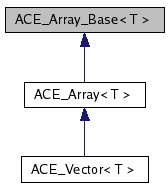# ACE_Array_Base< T > Class Template Reference

Implement a simple dynamic array. More...

`#include <Array_Base.h>`

Inheritance diagram for ACE_Array_Base< T >:[legend]
Collaboration diagram for ACE_Array_Base< T >:[legend]
List of all members.

## Public Types

typedef T TYPE
typedef ACE_Array_Iterator<
T >
ITERATOR

## Public Member Functions

ACE_Array_Base (size_t size=0, ACE_Allocator *the_allocator=0)
Dynamically create an uninitialized array.
ACE_Array_Base (size_t size, const T &default_value, ACE_Allocator *the_allocator=0)
Dynamically initialize the entire array to the <default_value>.
ACE_Array_Base (const ACE_Array_Base< T > &s)
void operator= (const ACE_Array_Base< T > &s)
~ACE_Array_Base (void)
Clean up the array (e.g., delete dynamically allocated memory).
T & operator[] (size_t slot)
const T & operator[] (size_t slot) const
int set (const T &new_item, size_t slot)
int get (T &item, size_t slot) const
size_t size (void) const
Returns the <cur_size_> of the array.
int size (size_t new_size)
size_t max_size (void) const
Returns the <max_size_> of the array.
int max_size (size_t new_size)

## Protected Member Functions

int in_range (size_t slot) const

## Protected Attributes

size_t max_size_
size_t cur_size_
T * array_
Pointer to the array's storage buffer.
ACE_Allocatorallocator_
Allocation strategy of the ACE_Array_Base.

## Friends

class ACE_Array_Iterator< T >

## Detailed Description

### template<class T> class ACE_Array_Base< T >

Implement a simple dynamic array.

This parametric class implements a simple dynamic array; resizing must be controlled by the user. No comparison or find operations are implemented.

## Member Typedef Documentation

template<class T>
 typedef ACE_Array_Iterator ACE_Array_Base< T >::ITERATOR

Reimplemented in ACE_Array< T >, ACE_Array< ACE_Get_Opt::ACE_Get_Opt_Long_Option * >, and ACE_Array< ACE_INET_Addr >.

template<class T>
 typedef T ACE_Array_Base< T >::TYPE

Reimplemented in ACE_Array< T >, ACE_Array< ACE_Get_Opt::ACE_Get_Opt_Long_Option * >, and ACE_Array< ACE_INET_Addr >.

## Constructor & Destructor Documentation

template<class T>
 ACE_BEGIN_VERSIONED_NAMESPACE_DECL ACE_Array_Base< T >::ACE_Array_Base ( size_t size = `0`, ACE_Allocator * the_allocator = `0` )

Dynamically create an uninitialized array.

template<class T>
 ACE_Array_Base< T >::ACE_Array_Base ( size_t size, const T & default_value, ACE_Allocator * the_allocator = `0` )

Dynamically initialize the entire array to the <default_value>.

template<class T>
 ACE_Array_Base< T >::ACE_Array_Base ( const ACE_Array_Base< T > & s )

The copy constructor performs initialization by making an exact copy of the contents of parameter <s>, i.e., *this == s will return true.

template<class T>
 ACE_BEGIN_VERSIONED_NAMESPACE_DECL ACE_INLINE ACE_Array_Base< T >::~ACE_Array_Base ( void )

Clean up the array (e.g., delete dynamically allocated memory).

## Member Function Documentation

template<class T>
 int ACE_Array_Base< T >::get ( T & item, size_t slot ) const

Get an item in the array at location <slot>. Returns -1 if <slot> is not in range, else returns 0. Note that this function copies the item. If you want to avoid the copy, you can use the const operator [], but then you'll be responsible for range checking.

template<class T>
 ACE_INLINE int ACE_Array_Base< T >::in_range ( size_t slot ) const` [protected]`

Returns 1 if <slot> is within range, i.e., 0 >= <slot> < <cur_size_>, else returns 0.

template<class T>
 int ACE_Array_Base< T >::max_size ( size_t new_size )

Changes the size of the array to match <new_size>. It copies the old contents into the new array. Return -1 on failure. It does not affect new_size

template<class T>
 ACE_INLINE size_t ACE_Array_Base< T >::max_size ( void ) const

Returns the <max_size_> of the array.

template<class T>
 void ACE_Array_Base< T >::operator= ( const ACE_Array_Base< T > & s )

Assignment operator performs an assignment by making an exact copy of the contents of parameter <s>, i.e., *this == s will return true. Note that if the <max_size_> of <array_> is >= than <s.max_size_> we can copy it without reallocating. However, if <max_size_> is < <s.max_size_> we must delete the <array_>, reallocate a new <array_>, and then copy the contents of <s>.

template<class T>
 ACE_INLINE const T & ACE_Array_Base< T >::operator[] ( size_t slot ) const

Get item in the array at location <slot>. Doesn't perform range checking.

template<class T>
 ACE_INLINE T & ACE_Array_Base< T >::operator[] ( size_t slot )

Set item in the array at location <slot>. Doesn't perform range checking.

template<class T>
 int ACE_Array_Base< T >::set ( const T & new_item, size_t slot )

Set an item in the array at location <slot>. Returns -1 if <slot> is not in range, else returns 0.

template<class T>
 int ACE_Array_Base< T >::size ( size_t new_size )

Changes the size of the array to match <new_size>. It copies the old contents into the new array. Return -1 on failure.

template<class T>
 ACE_INLINE size_t ACE_Array_Base< T >::size ( void ) const

Returns the <cur_size_> of the array.

Reimplemented in ACE_Vector< T, DEFAULT_SIZE >.

## Friends And Related Function Documentation

template<class T>
 friend class ACE_Array_Iterator< T >` [friend]`

## Member Data Documentation

template<class T>
 ACE_Allocator* ACE_Array_Base< T >::allocator_` [protected]`

Allocation strategy of the ACE_Array_Base.

template<class T>
 T* ACE_Array_Base< T >::array_` [protected]`

Pointer to the array's storage buffer.

template<class T>
 size_t ACE_Array_Base< T >::cur_size_` [protected]`

Current size of the array. This starts out being == to <max_size_>. However, if we are assigned a smaller array, then <cur_size_> will become less than <max_size_>. The purpose of keeping track of both sizes is to avoid reallocating memory if we don't have to.

template<class T>
 size_t ACE_Array_Base< T >::max_size_` [protected]`

Maximum size of the array, i.e., the total number of <T> elements in <array_>.

The documentation for this class was generated from the following files:
Generated on Sun Jul 9 09:21:44 2006 for ACE by1.4.7-1